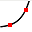Enable text based alternatives for graph display and drawing entry

Try Another Version of This Question

Graph the Exponential Function f(x) = 4(1/4)^x by plotting the Vertical Intercept and one other point. Then write the Vertical Intercept as an ordered pair.
Clear All Draw:The Vertical Intercept is:

Get help: